# Floquet calibration

This notebook demonstrates the Floquet calibration API, a tool for characterizing $\sqrt{\text{iSWAP} }$ gates and inserting single-qubit $Z$ phases to compensate for errors. This characterization is done by the Quantum Engine and the insertion of $Z$ phases for compensation/calibration is completely client-side with the help of Cirq utilities. At the highest level, the tool inputs a quantum circuit of interest (as well as a backend to run on) and outputs a calibrated circuit for this backend which can then be executed to produce better results.

## Details on the calibration tool

In more detail, assuming we have a number-convserving two-qubit unitary gate, Floquet calibration (FC) returns fast, accurate estimates for the relevant angles to be calibrated. The cirq.PhasedFSimGate has five angles $\theta$, $\zeta$, $\chi$, $\gamma$, $\phi$ with unitary matrix

$$\left[ \begin{matrix} 1 & 0 & 0 & 0 \\ 0 & \exp(-i \gamma - i \zeta) cos( \theta ) & -i \exp(-i \gamma + i \chi) sin( \theta ) & 0 \\ 0 & -i \exp(-i \gamma - i \chi) sin( \theta ) & \exp(-i \gamma + i \zeta) cos( \theta) & 0 \\ 0 & 0 & 0 & \exp(-2 i \gamma -i \phi ) \end{matrix} \right]$$

With Floquet calibration, every angle but $\chi$ can be calibrated. In experiments, we have found these angles change when gates are run in parallel. Because of this, we perform FC on entire moments of two-qubits gates and return different characterized angles for each.

After characterizing a set of angles, one needs to adjust the circuit to compensate for the offset. The simplest adjustment is for $\zeta$ and $\gamma$ and works by adding $R_z$ gates before and after the two-qubit gates in question. For many circuits, even this simplest compensation can lead to a significant improvement in results. We provide methods for doing this in this notebook and analyze results for an example circuit.

We do not attempt to correct the misaligned iSWAP rotation or the additional two-qubit phase in this notebook. This is a non-trivial task and we do currently have simple tools to achieve this. It is up to the user to correct for these as best as possible.

## Setup

try:
import cirq
except ImportError:
print("installing cirq...")
!pip install cirq --quiet
print("installed cirq.")

from typing import Iterable, List, Optional, Sequence

import matplotlib.pyplot as plt
import numpy as np
import os

import cirq
import cirq_google as cg  # Contains the Floquet calibration tools.


Running the next cell will prompt you to authenticate Google Cloud SDK to use your project. See the Getting Started Guide for more information.

# The Google Cloud Project id to use.
project_id = ''

if project_id == '' and 'GOOGLE_CLOUD_PROJECT' not in os.environ:
print("No project_id provided and environment variable "
"GOOGLE_CLOUD_PROJECT not set, defaulting to noisy simulator.")
sampler = cg.PhasedFSimEngineSimulator.create_with_random_gaussian_sqrt_iswap(
mean=cg.SQRT_ISWAP_INV_PARAMETERS,
sigma=cg.PhasedFSimCharacterization(
theta=0.01, zeta=0.10, chi=0.01, gamma=0.10, phi=0.02
),
)
device = cg.Bristlecone
line_length = 20
else:
import os
os.environ['GOOGLE_CLOUD_PROJECT'] = project_id

def authenticate_user():
"""Runs the user through the Colab OAuth process.

Checks for Google Application Default Credentials and runs interactive login
if the notebook is executed in Colab. In case the notebook is executed in Jupyter notebook
or other IPython runtimes, no interactive login is provided, it is assumed that the
GOOGLE_APPLICATION_CREDENTIALS env var is set or gcloud auth application-default login
was executed already.

For more information on using Application Default Credentials see
https://cloud.google.com/docs/authentication/production
"""
in_colab = False
try:
from IPython import get_ipython
in_colab = 'google.colab' in str(get_ipython())
except:
# Notebook is not executed within IPython. Assuming external authentication.
return

if in_colab:
from google.colab import auth
print("Getting OAuth2 credentials.")
print("Press enter after entering the verification code.")
auth.authenticate_user(clear_output=False)
print("Authentication complete.")
else:
print("Notebook is not executed with Colab, assuming Application Default Credentials are setup.")

authenticate_user()
print("Successful authentication to Google Cloud.")

processor_id = ""
engine = cg.get_engine()
device = cg.get_engine_device(processor_id)
sampler = cg.get_engine_sampler(processor_id, gate_set_name="sqrt_iswap")
line_length = 35

No project_id provided and environment variable GOOGLE_CLOUD_PROJECT not set, defaulting to noisy simulator.


## Minimal example for a single $\sqrt{\text{iSWAP} }$ gate

To see how the API is used, we first show the simplest usage of Floquet calibration for a minimal example of one $\sqrt{\text{iSWAP} }$ gate. After this section, we show detailed usage with a larger circuit and analyze the results.

The gates that are calibrated by Floquet calibration are $\sqrt{\text{iSWAP} }$ gates:

sqrt_iswap = cirq.FSimGate(np.pi / 4, 0.0)
print(cirq.unitary(sqrt_iswap).round(3))

[[1.   +0.j    0.   +0.j    0.   +0.j    0.   +0.j   ]
[0.   +0.j    0.707+0.j    0.   -0.707j 0.   +0.j   ]
[0.   +0.j    0.   -0.707j 0.707+0.j    0.   +0.j   ]
[0.   +0.j    0.   +0.j    0.   +0.j    1.   -0.j   ]]


First we get two connected qubits on the selected device and define a circuit.

"""Define a simple circuit to use Floquet calibration on."""
qubits = cg.line_on_device(device, length=2)
circuit = cirq.Circuit(sqrt_iswap.on(*qubits))

# Display it.
print("Circuit to calibrate:\n")
print(circuit)

Circuit to calibrate:

(0, 5): ───FSim(0.25π, 0)───
│
(0, 6): ───FSim(0.25π, 0)───


The simplest way to use Floquet calibration is as follows.

"""Simplest usage of Floquet calibration."""
calibrated_circuit, *_ = cg.run_zeta_chi_gamma_compensation_for_moments(
circuit,
sampler
)


When we print out the returned calibrated_circuit.circuit below, we see the added $Z$ rotations to compensate for errors.

print("Calibrated circuit:\n")
calibrated_circuit.circuit

Calibrated circuit:


This calibrated_circuit can now be executed on the processor to produce better results.

## More detailed example with a larger circuit

We now use Floquet calibration on a larger circuit which models the evolution of a fermionic particle on a linear spin chain. The physics of this problem for a closed chain (here we use an open chain) has been studied in Accurately computing electronic properties of materials using eigenenergies, but for the purposes of this notebook we can treat this just as an example to demonstrate Floquet calibration on.

First we use the function cirq_google.line_on_device to return a line of qubits of a specified length.

line = cg.line_on_device(device, line_length)
print(line)

(0, 5)━━(0, 6)
┃
(1, 6)━━(1, 7)
┃
(2, 6)  (2, 7)━━(2, 8)
┃               ┃
(3, 6)━━(3, 7)  (3, 8)━━(3, 9)
┃               ┃
(4, 7)━━(4, 8)  (4, 9)━━(4, 10)
┃               ┃
(5, 8)━━(5, 9)  (5, 10)
┃       ┃
(6, 9)━━(6, 10)


This line is now broken up into a number of segments of a specified length (number of qubits).

segment_length = 5
segments = [line[i: i + segment_length]
for i in range(0, line_length - segment_length + 1, segment_length)]


For example, the first segment consists of the following qubits.

print(*segments)

(0, 5) (0, 6) (1, 6) (1, 7) (2, 7)


We now implement a number of Trotter steps on each segment in parallel. The middle qubit on each segment is put into the $|1\rangle$ state, then each Trotter step consists of staggered $\sqrt{\text{iSWAP} }$ gates. All qubits are measured in the $Z$ basis at the end of the circuit.

For convenience, this code is wrapped in a function.

def create_example_circuit(
segments: Sequence[Sequence[cirq.Qid]],
num_trotter_steps: int,
) -> cirq.Circuit:
"""Returns a linear chain circuit to demonstrate Floquet calibration on."""
circuit = cirq.Circuit()

# Initial state preparation.
for segment in segments:
circuit += [cirq.X.on(segment[len(segment) // 2])]

# Trotter steps.
for step in range(num_trotter_steps):
offset = step % 2
moment = cirq.Moment()
for segment in segments:
moment += cirq.Moment(
[sqrt_iswap.on(a, b) for a, b in zip(segment[offset::2],
segment[offset + 1::2])])
circuit += moment

# Measurement.
circuit += cirq.measure(*sum(segments, ()), key='z')
return circuit


As an example, we show this circuit on the first segment of the line from above.

"""Example of the linear chain circuit on one segment of the line."""
num_trotter_steps = 20

circuit_on_segment = create_example_circuit(
segments=[segments],
num_trotter_steps=num_trotter_steps,
)
print(circuit_on_segment.to_text_diagram(qubit_order=segments))

(0, 5): ───────FSim(0.25π, 0)────────────────────FSim(0.25π, 0)────────────────────FSim(0.25π, 0)────────────────────FSim(0.25π, 0)────────────────────FSim(0.25π, 0)────────────────────FSim(0.25π, 0)────────────────────FSim(0.25π, 0)────────────────────FSim(0.25π, 0)────────────────────FSim(0.25π, 0)────────────────────FSim(0.25π, 0)────────────────────M('z')───
│                                 │                                 │                                 │                                 │                                 │                                 │                                 │                                 │                                 │                                 │
(0, 6): ───────FSim(0.25π, 0)───FSim(0.25π, 0)───FSim(0.25π, 0)───FSim(0.25π, 0)───FSim(0.25π, 0)───FSim(0.25π, 0)───FSim(0.25π, 0)───FSim(0.25π, 0)───FSim(0.25π, 0)───FSim(0.25π, 0)───FSim(0.25π, 0)───FSim(0.25π, 0)───FSim(0.25π, 0)───FSim(0.25π, 0)───FSim(0.25π, 0)───FSim(0.25π, 0)───FSim(0.25π, 0)───FSim(0.25π, 0)───FSim(0.25π, 0)───FSim(0.25π, 0)───M────────
│                                 │                                 │                                 │                                 │                                 │                                 │                                 │                                 │                                 │                │
(1, 6): ───X───FSim(0.25π, 0)───FSim(0.25π, 0)───FSim(0.25π, 0)───FSim(0.25π, 0)───FSim(0.25π, 0)───FSim(0.25π, 0)───FSim(0.25π, 0)───FSim(0.25π, 0)───FSim(0.25π, 0)───FSim(0.25π, 0)───FSim(0.25π, 0)───FSim(0.25π, 0)───FSim(0.25π, 0)───FSim(0.25π, 0)───FSim(0.25π, 0)───FSim(0.25π, 0)───FSim(0.25π, 0)───FSim(0.25π, 0)───FSim(0.25π, 0)───FSim(0.25π, 0)───M────────
│                                 │                                 │                                 │                                 │                                 │                                 │                                 │                                 │                                 │                                 │
(1, 7): ───────FSim(0.25π, 0)───FSim(0.25π, 0)───FSim(0.25π, 0)───FSim(0.25π, 0)───FSim(0.25π, 0)───FSim(0.25π, 0)───FSim(0.25π, 0)───FSim(0.25π, 0)───FSim(0.25π, 0)───FSim(0.25π, 0)───FSim(0.25π, 0)───FSim(0.25π, 0)───FSim(0.25π, 0)───FSim(0.25π, 0)───FSim(0.25π, 0)───FSim(0.25π, 0)───FSim(0.25π, 0)───FSim(0.25π, 0)───FSim(0.25π, 0)───FSim(0.25π, 0)───M────────
│                                 │                                 │                                 │                                 │                                 │                                 │                                 │                                 │                                 │                │
(2, 7): ────────────────────────FSim(0.25π, 0)────────────────────FSim(0.25π, 0)────────────────────FSim(0.25π, 0)────────────────────FSim(0.25π, 0)────────────────────FSim(0.25π, 0)────────────────────FSim(0.25π, 0)────────────────────FSim(0.25π, 0)────────────────────FSim(0.25π, 0)────────────────────FSim(0.25π, 0)────────────────────FSim(0.25π, 0)───M────────


The circuit we will use for Floquet calibration is this same pattern repeated on all segments of the line.

"""Circuit used to demonstrate Floquet calibration."""
circuit = create_example_circuit(
segments=segments,
num_trotter_steps=num_trotter_steps
)


### Execution on a simulator

To establish a "ground truth," we first simulate a segment on a noiseless simulator.

"""Simulate one segment on a simulator."""
nreps = 20_000
sim_result = cirq.Simulator().run(circuit_on_segment, repetitions=nreps)


### Execution on the processor without Floquet calibration

We now execute the full circuit on a processor without using Floquet calibration.

"""Execute the full circuit on a processor without Floquet calibration."""
raw_results = sampler.run(circuit, repetitions=nreps)


### Comparing raw results to simulator results

For comparison we will plot densities (average measurement results) on each segment. Such densities are in the interval $[0, 1]$ and more accurate results are closer to the simulator results.

To visualize results, we define a few helper functions.

#### Helper functions

The next cell defines two functions for returning the density (average measurement results) on a segment or on all segments. We can optionally post-select for measurements with a specific filling (particle number) - i.e., discard measurement results which don't obey this expected particle number.

def z_density_from_measurements(
measurements: np.ndarray,
post_select_filling: Optional[int] = 1
) -> np.ndarray:
"""Returns density for one segment on the line."""
counts = np.sum(measurements, axis=1, dtype=int)

if post_select_filling is not None:
errors = np.abs(counts - post_select_filling)
counts = measurements[errors == 0]

return np.average(counts, axis=0)

def z_densities_from_result(
result: cirq.Result,
segments: Iterable[Sequence[cirq.Qid]],
post_select_filling: Optional[int] = 1
) -> List[np.ndarray]:
"""Returns densities for each segment on the line."""
measurements = result.measurements['z']
z_densities = []

offset = 0
for segment in segments:
z_densities.append(z_density_from_measurements(
measurements[:, offset: offset + len(segment)],
post_select_filling)
)
offset += len(segment)
return z_densities


Now we define functions to plot the densities for the simulator, processor without Floquet calibration, and processor with Floquet calibration (which we will use at the end of this notebook). The first function is for a single segment, and the second function is for all segments.

def plot_density(
ax: plt.Axes,
sim_density: np.ndarray,
raw_density: np.ndarray,
cal_density: Optional[np.ndarray] = None,
raw_errors: Optional[np.ndarray] = None,
cal_errors: Optional[np.ndarray] = None,
title: Optional[str] = None,
show_legend: bool = True,
show_ylabel: bool = True,
) -> None:
"""Plots the density of a single segment for simulated, raw, and calibrated
results.
"""
colors = ["grey", "orange", "green"]
alphas = [0.5, 0.8, 0.8]
labels = ["sim", "raw", "cal"]

# Plot densities.
for i, density in enumerate([sim_density, raw_density, cal_density]):
if density is not None:
ax.plot(
range(len(density)),
density,
"-o" if i == 0 else "o",
markersize=11,
color=colors[i],
alpha=alphas[i],
label=labels[i]
)

# Plot errors if provided.
errors = [raw_errors, cal_errors]
densities = [raw_density, cal_density]
for i, (errs, dens) in enumerate(zip(errors, densities)):
if errs is not None:
ax.errorbar(
range(len(errs)),
dens,
errs,
linestyle='',
color=colors[i + 1],
capsize=8,
elinewidth=2,
markeredgewidth=2
)

# Titles, axes, and legend.
ax.set_xticks(list(range(len(sim_density))))
ax.set_xlabel("Qubit index in segment")
if show_ylabel:
ax.set_ylabel("Density")
if title:
ax.set_title(title)
if show_legend:
ax.legend()

def plot_densities(
sim_density: np.ndarray,
raw_densities: Sequence[np.ndarray],
cal_densities: Optional[Sequence[np.ndarray]] = None,
rows: int = 3
) -> None:
"""Plots densities for simulated, raw, and calibrated results on all segments.
"""
if not cal_densities:
cal_densities = [None] * len(raw_densities)

cols = (len(raw_densities) + rows - 1) // rows

fig, axes = plt.subplots(
rows, cols, figsize=(cols * 4, rows * 3.5), sharey=True
)
if rows == 1 and cols == 1:
axes = [axes]
elif rows > 1 and cols > 1:
axes = [axes[row, col] for row in range(rows) for col in range(cols)]

for i, (ax, raw, cal) in enumerate(zip(axes, raw_densities, cal_densities)):
plot_density(
ax,
sim_density,
raw,
cal,
title=f"Segment {i + 1}",
show_legend=False,
show_ylabel=i % cols == 0
)

# Common legend for all subplots.
handles, labels = ax.get_legend_handles_labels()
fig.legend(handles, labels)

plt.tight_layout(pad=0.1, w_pad=1.0, h_pad=3.0)


#### Visualizing results

To visualize results, we first extract densities from the measurements.

"""Extract densities from measurement results."""
# Simulator density.
sim_density, = z_densities_from_result(sim_result,[circuit_on_segment])

# Processor densities without Floquet calibration.
raw_densities = z_densities_from_result(raw_results, segments)


We first plot the densities on each segment. Note that the simulator densities ("sim") are repeated on each segment and the lines connecting them are just visual guides.

plot_densities(sim_density, raw_densities, rows=int(np.sqrt(line_length / segment_length)))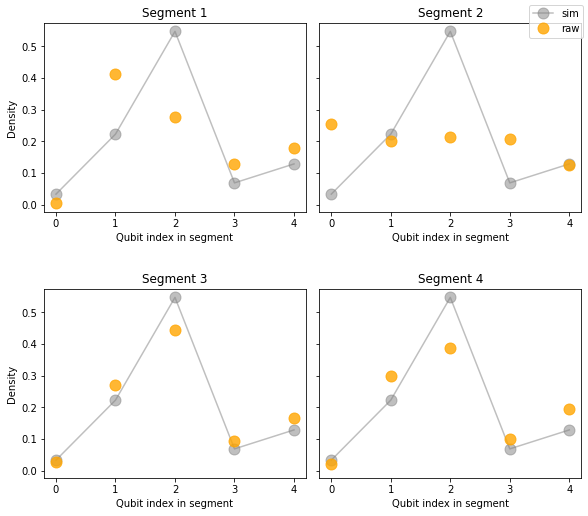We can also look at the average and variance over the segments.

"""Plot mean density and variance over segments."""
raw_avg = np.average(raw_densities, axis=0)
raw_std = np.std(raw_densities, axis=0, ddof=1)

plot_density(
plt.gca(),
sim_density,
raw_density=raw_avg,
raw_errors=raw_std,
title="Average over segments"
)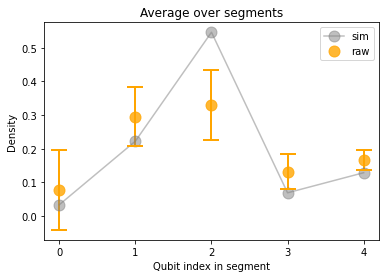In the next section, we will use Floquet calibration to produce better average results. After running the circuit with Floquet calibration, we will use these same visualizations to compare results.

### Execution on the processor with Floquet calibration

There are two equivalent ways to use Floquet calibration which we outline below. A rough estimate for the time required for Floquet calibration is about 16 seconds per 10 qubits, plus 30 seconds of overhead, per calibrated moment.

#### Simple usage

The first way to use Floquet calibration is via the single function call used at the start of this notebook. Here, we describe the remaining returned values in addition to calibrated_circuit.

# (calibrated_circuit, calibrations
#  ) = cg.run_zeta_chi_gamma_compensation_for_moments(
#     circuit,
#     sampler,
# )


The returned calibrated_circuit.circuit can then be run on the engine. The full list of returned arguments is as follows:

• calibrated_circuit.circuit: The input circuit with added $Z$ rotations around each $\sqrt{\text{iSWAP} }$ gate to compensate for errors.
• calibrated_circuit.moment_to_calibration: Provides an index of the matching characterization (index in calibrations list) for each moment of the calibrated_circuit.circuit, or None if the moment was not characterized (e.g., for a measurement outcome).
• calibrations: List of characterization results for each characterized moment. Each characterization contains angles for each qubit pair.

#### Step-by-step usage

The above function cirq_google.run_zeta_chi_gamma_compensation_for_moments performs the following three steps:

1. Find moments within the circuit that need to be characterized.
2. Characterize them on the engine.
3. Apply corrections to the original circuit.

To find moments that need to be characterized, we can do the following.

"""Step 1: Find moments in the circuit that need to be characterized."""
(characterized_circuit, characterization_requests
) = cg.prepare_characterization_for_moments(
circuit,
options=cg.FloquetPhasedFSimCalibrationOptions(
characterize_theta=False,
characterize_zeta=True,
characterize_chi=False,
characterize_gamma=True,
characterize_phi=False
)
)


The characterization_requests contain information on the operations (gate + qubit pairs) to characterize.

"""Show an example characterization request."""
print(f"Total {len(characterization_requests)} moment(s) to characterize.")

print("\nExample request")
request = characterization_requests
print("Gate:", request.gate)
print("Qubit pairs:", request.pairs)
print("Options: ", request.options)

Total 2 moment(s) to characterize.

Example request
Gate: cirq.FSimGate(theta=0.7853981633974483, phi=0.0)
Qubit pairs: ((cirq.GridQubit(0, 5), cirq.GridQubit(0, 6)), (cirq.GridQubit(1, 6), cirq.GridQubit(1, 7)), (cirq.GridQubit(2, 8), cirq.GridQubit(3, 8)), (cirq.GridQubit(3, 6), cirq.GridQubit(3, 7)), (cirq.GridQubit(3, 9), cirq.GridQubit(4, 9)), (cirq.GridQubit(4, 7), cirq.GridQubit(4, 8)), (cirq.GridQubit(5, 9), cirq.GridQubit(6, 9)), (cirq.GridQubit(5, 10), cirq.GridQubit(6, 10)))
Options:  FloquetPhasedFSimCalibrationOptions(characterize_theta=False, characterize_zeta=True, characterize_chi=False, characterize_gamma=True, characterize_phi=False, readout_error_tolerance=None)


We now characterize them on the engine using cirq_google.run_calibrations.

"""Step 2: Characterize moments on the engine."""
characterizations = cg.run_calibrations(
characterization_requests,
sampler,
max_layers_per_request=1,
)


The characterizations store characterization results for each pair in each moment, for example.

print(f"Total: {len(characterizations)} characterizations.")
print()

(pair, parameters), *_ = characterizations.parameters.items()
print(f"Example pair: {pair}")
print(f"Example parameters: {parameters}")

Total: 2 characterizations.

Example pair: (cirq.GridQubit(0, 5), cirq.GridQubit(0, 6))
Example parameters: PhasedFSimCharacterization(theta=None, zeta=0.08087884235445279, chi=None, gamma=-0.062349164375367486, phi=None)


Finally, we apply corrections to the original circuit.

"""Step 3: Apply corrections to the circuit to get a calibrated circuit."""
calibrated_circuit = cg.make_zeta_chi_gamma_compensation_for_moments(
characterized_circuit,
characterizations
)


The calibrated circuit can now be run on the processor. We first inspect the calibrated circuit to compare to the original.

print("Portion of calibrated circuit:")
print("\n".join(
calibrated_circuit.circuit.to_text_diagram(qubit_order=line).splitlines()[:9] +
["..."]))

Portion of calibrated circuit:
(0, 5): ────────Rz(-0.023π)───FSim(0.25π, 0)───Rz(-0.023π)────────────────────────────────────────────────Rz(-0.023π)───FSim(0.25π, 0)───Rz(-0.023π)────────────────────────────────────────────────Rz(-0.023π)───FSim(0.25π, 0)───Rz(-0.023π)────────────────────────────────────────────────Rz(-0.023π)───FSim(0.25π, 0)───Rz(-0.023π)────────────────────────────────────────────────Rz(-0.023π)───FSim(0.25π, 0)───Rz(-0.023π)────────────────────────────────────────────────Rz(-0.023π)───FSim(0.25π, 0)───Rz(-0.023π)────────────────────────────────────────────────Rz(-0.023π)───FSim(0.25π, 0)───Rz(-0.023π)────────────────────────────────────────────────Rz(-0.023π)───FSim(0.25π, 0)───Rz(-0.023π)────────────────────────────────────────────────Rz(-0.023π)───FSim(0.25π, 0)───Rz(-0.023π)────────────────────────────────────────────────Rz(-0.023π)───FSim(0.25π, 0)───Rz(-0.023π)────────────────────────────────────────────────M('z')───
│                                                                                         │                                                                                         │                                                                                         │                                                                                         │                                                                                         │                                                                                         │                                                                                         │                                                                                         │                                                                                         │                                                                           │
(0, 6): ────────Rz(0.003π)────FSim(0.25π, 0)───Rz(0.003π)────Rz(-0.014π)───FSim(0.25π, 0)───Rz(-0.014π)───Rz(0.003π)────FSim(0.25π, 0)───Rz(0.003π)────Rz(-0.014π)───FSim(0.25π, 0)───Rz(-0.014π)───Rz(0.003π)────FSim(0.25π, 0)───Rz(0.003π)────Rz(-0.014π)───FSim(0.25π, 0)───Rz(-0.014π)───Rz(0.003π)────FSim(0.25π, 0)───Rz(0.003π)────Rz(-0.014π)───FSim(0.25π, 0)───Rz(-0.014π)───Rz(0.003π)────FSim(0.25π, 0)───Rz(0.003π)────Rz(-0.014π)───FSim(0.25π, 0)───Rz(-0.014π)───Rz(0.003π)────FSim(0.25π, 0)───Rz(0.003π)────Rz(-0.014π)───FSim(0.25π, 0)───Rz(-0.014π)───Rz(0.003π)────FSim(0.25π, 0)───Rz(0.003π)────Rz(-0.014π)───FSim(0.25π, 0)───Rz(-0.014π)───Rz(0.003π)────FSim(0.25π, 0)───Rz(0.003π)────Rz(-0.014π)───FSim(0.25π, 0)───Rz(-0.014π)───Rz(0.003π)────FSim(0.25π, 0)───Rz(0.003π)────Rz(-0.014π)───FSim(0.25π, 0)───Rz(-0.014π)───Rz(0.003π)────FSim(0.25π, 0)───Rz(0.003π)────Rz(-0.014π)───FSim(0.25π, 0)───Rz(-0.014π)───M────────
│                                                                                         │                                                                                         │                                                                                         │                                                                                         │                                                                                         │                                                                                         │                                                                                         │                                                                                         │                                                                                         │                              │
(1, 6): ────X───Rz(0.044π)────FSim(0.25π, 0)───Rz(0.044π)────Rz(0.027π)────FSim(0.25π, 0)───Rz(0.027π)────Rz(0.044π)────FSim(0.25π, 0)───Rz(0.044π)────Rz(0.027π)────FSim(0.25π, 0)───Rz(0.027π)────Rz(0.044π)────FSim(0.25π, 0)───Rz(0.044π)────Rz(0.027π)────FSim(0.25π, 0)───Rz(0.027π)────Rz(0.044π)────FSim(0.25π, 0)───Rz(0.044π)────Rz(0.027π)────FSim(0.25π, 0)───Rz(0.027π)────Rz(0.044π)────FSim(0.25π, 0)───Rz(0.044π)────Rz(0.027π)────FSim(0.25π, 0)───Rz(0.027π)────Rz(0.044π)────FSim(0.25π, 0)───Rz(0.044π)────Rz(0.027π)────FSim(0.25π, 0)───Rz(0.027π)────Rz(0.044π)────FSim(0.25π, 0)───Rz(0.044π)────Rz(0.027π)────FSim(0.25π, 0)───Rz(0.027π)────Rz(0.044π)────FSim(0.25π, 0)───Rz(0.044π)────Rz(0.027π)────FSim(0.25π, 0)───Rz(0.027π)────Rz(0.044π)────FSim(0.25π, 0)───Rz(0.044π)────Rz(0.027π)────FSim(0.25π, 0)───Rz(0.027π)────Rz(0.044π)────FSim(0.25π, 0)───Rz(0.044π)────Rz(0.027π)────FSim(0.25π, 0)───Rz(0.027π)────M────────
│                                                                                         │                                                                                         │                                                                                         │                                                                                         │                                                                                         │                                                                                         │                                                                                         │                                                                                         │                                                                                         │                                                                           │
(1, 7): ────────Rz(0.004π)────FSim(0.25π, 0)───Rz(0.004π)────Rz(-0.002π)───FSim(0.25π, 0)───Rz(-0.002π)───Rz(0.004π)────FSim(0.25π, 0)───Rz(0.004π)────Rz(-0.002π)───FSim(0.25π, 0)───Rz(-0.002π)───Rz(0.004π)────FSim(0.25π, 0)───Rz(0.004π)────Rz(-0.002π)───FSim(0.25π, 0)───Rz(-0.002π)───Rz(0.004π)────FSim(0.25π, 0)───Rz(0.004π)────Rz(-0.002π)───FSim(0.25π, 0)───Rz(-0.002π)───Rz(0.004π)────FSim(0.25π, 0)───Rz(0.004π)────Rz(-0.002π)───FSim(0.25π, 0)───Rz(-0.002π)───Rz(0.004π)────FSim(0.25π, 0)───Rz(0.004π)────Rz(-0.002π)───FSim(0.25π, 0)───Rz(-0.002π)───Rz(0.004π)────FSim(0.25π, 0)───Rz(0.004π)────Rz(-0.002π)───FSim(0.25π, 0)───Rz(-0.002π)───Rz(0.004π)────FSim(0.25π, 0)───Rz(0.004π)────Rz(-0.002π)───FSim(0.25π, 0)───Rz(-0.002π)───Rz(0.004π)────FSim(0.25π, 0)───Rz(0.004π)────Rz(-0.002π)───FSim(0.25π, 0)───Rz(-0.002π)───Rz(0.004π)────FSim(0.25π, 0)───Rz(0.004π)────Rz(-0.002π)───FSim(0.25π, 0)───Rz(-0.002π)───M────────
│                                                                                         │                                                                                         │                                                                                         │                                                                                         │                                                                                         │                                                                                         │                                                                                         │                                                                                         │                                                                                         │                              │
(2, 7): ─────────────────────────────────────────────────────Rz(-0.018π)───FSim(0.25π, 0)───Rz(-0.018π)────────────────────────────────────────────────Rz(-0.018π)───FSim(0.25π, 0)───Rz(-0.018π)────────────────────────────────────────────────Rz(-0.018π)───FSim(0.25π, 0)───Rz(-0.018π)────────────────────────────────────────────────Rz(-0.018π)───FSim(0.25π, 0)───Rz(-0.018π)────────────────────────────────────────────────Rz(-0.018π)───FSim(0.25π, 0)───Rz(-0.018π)────────────────────────────────────────────────Rz(-0.018π)───FSim(0.25π, 0)───Rz(-0.018π)────────────────────────────────────────────────Rz(-0.018π)───FSim(0.25π, 0)───Rz(-0.018π)────────────────────────────────────────────────Rz(-0.018π)───FSim(0.25π, 0)───Rz(-0.018π)────────────────────────────────────────────────Rz(-0.018π)───FSim(0.25π, 0)───Rz(-0.018π)────────────────────────────────────────────────Rz(-0.018π)───FSim(0.25π, 0)───Rz(-0.018π)───M────────
...


Note again that $\sqrt{\text{iSWAP} }$ gates are padded by $Z$ phases to compensate for errors. We now run this calibrated circuit.

"""Run the calibrated circuit on the engine."""
cal_results = sampler.run(calibrated_circuit.circuit, repetitions=nreps)


### Comparing raw results to calibrated results

We now compare results with and without Floquet calibration, again using the simulator results as a baseline for comparison. First we extract the calibrated densities.

"""Extract densities from measurement results."""
cal_densities = z_densities_from_result(cal_results, segments)


Now we reproduce the same density plots from above on each segment, this time including the calibrated ("cal") results.

plot_densities(
sim_density, raw_densities, cal_densities, rows=int(np.sqrt(line_length / segment_length))
)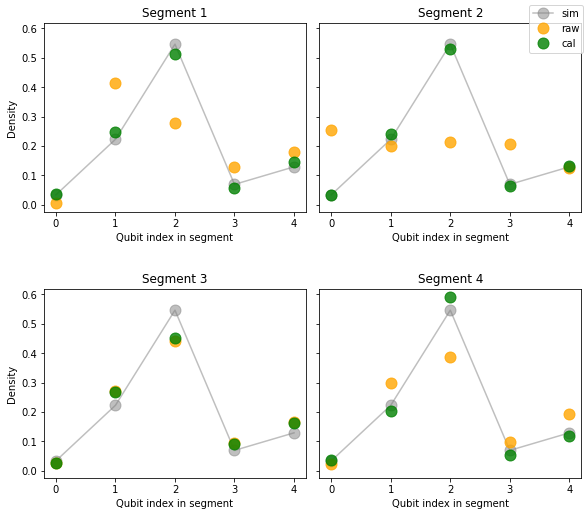We also visualize the mean and variance of results over segments as before.

"""Plot mean density and variance over segments."""
raw_avg = np.average(raw_densities, axis=0)
raw_std = np.std(raw_densities, axis=0, ddof=1)

cal_avg = np.average(cal_densities, axis=0)
cal_std = np.std(cal_densities, axis=0, ddof=1)

plot_density(
plt.gca(),
sim_density,
raw_avg,
cal_avg,
raw_std,
cal_std,
title="Average over segments"
)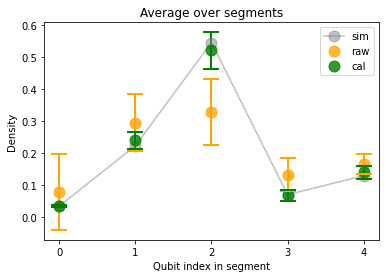Last, we can look at density errors between raw/calibrated results and simulated results.

"""Plot errors of raw vs calibrated results."""
fig, axes = plt.subplots(ncols=2, figsize=(15, 4))

axes.set_title("Error of the mean")
axes.set_ylabel("Density")
axes.set_title("Data standard deviation")

colors = ["orange", "green"]
labels = ["raw", "cal"]

for index, density in enumerate([raw_densities, cal_densities]):
color = colors[index]
label = labels[index]

average_density = np.average(density, axis=0)
sites = list(range(len(average_density)))

error = np.abs(average_density - sim_density)
std_dev = np.std(density, axis=0, ddof=1)

axes.plot(sites, error, color=color, alpha=0.6)
axes.scatter(sites, error, color=color)

axes.plot(sites, std_dev, label=label, color=color, alpha=0.6)
axes.scatter(sites, std_dev, color=color)

for ax in axes:
ax.set_xticks(sites)
ax.set_xlabel("Qubit index in segment")

plt.legend();

<matplotlib.legend.Legend at 0x7fb695f4d710>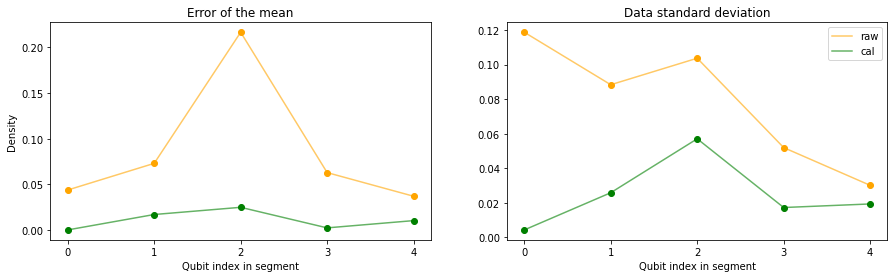[{ "type": "thumb-down", "id": "missingTheInformationINeed", "label":"Missing the information I need" },{ "type": "thumb-down", "id": "tooComplicatedTooManySteps", "label":"Too complicated / too many steps" },{ "type": "thumb-down", "id": "outOfDate", "label":"Out of date" },{ "type": "thumb-down", "id": "samplesCodeIssue", "label":"Samples / code issue" },{ "type": "thumb-down", "id": "otherDown", "label":"Other" }]
[{ "type": "thumb-up", "id": "easyToUnderstand", "label":"Easy to understand" },{ "type": "thumb-up", "id": "solvedMyProblem", "label":"Solved my problem" },{ "type": "thumb-up", "id": "otherUp", "label":"Other" }]